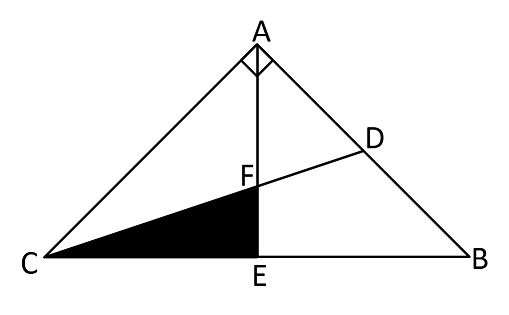# Isosceles Right-angled Triangle

Geometry Level 2ABC is a right triangle as shown. Given that $D$ is the midpoint of $AB$, $E$ is the midpoint of $BC$, and the side lengths of $AB$ and $AC$ are both 12, find the area of the shaded region.

×